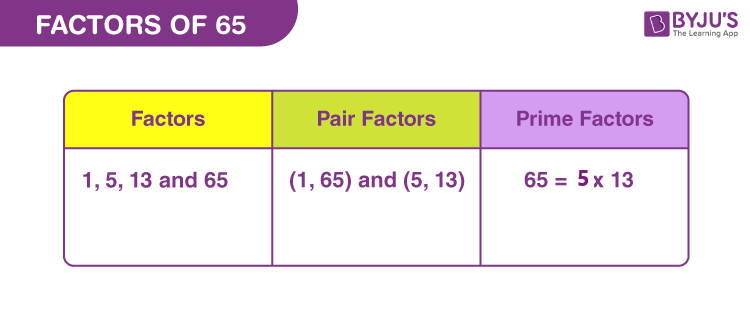# Factors of 65

In Mathematics, factors of 65 are the natural numbers that can divide the original number, wholly. When two natural numbers are multiplied together and they result in 65, then these numbers are called factors. 65 has a total four factors, therefore it is called a composite number.

Factors break the number into equal parts. 65 is always divisible by its factors. Thus, 65 divided by any of its factors, will result in a whole number and the remainder is 0. Let us find the factors in this article.## How to Find the Factors of 65?

Factors of 65 divide the original number, exactly. We know all the numbers are the factors of themselves and also 1 is the factor of all the numbers. Therefore, the two definite factors of 65 are 1 and 65. Now, let us find the other factors.

65 ÷ 1 = 65

65 ÷ 5 = 13

65 ÷ 13 = 5

65 ÷ 65 = 1

Therefore, the factors of 65 are 1, 5, 13 and 65.

## Pair Factors of 65

If the product of two integers is 65, then the pair of integers are called pair factors of 65. One definite pair is 1 and the number itself, i.e., (1, 65).

1 × 65 = 65

5 × 13 = 65

Therefore, the pair factors are (1, 65) and (5, 13).

We can also write the pair factors in negative terms, since the product of two negative factors will result in a positive number (i.e., original number).

-1 × -65 = 65

-5 × -13 = 65

Therefore, the negative pair factors are (-1, -65), and (-5, -13).

## Prime Factorisation of 65

Prime factorisation of 65 gives the product of prime numbers that will be equal to the original number.Here, we need to find the prime numbers that can completely divide the original number. To find these prime factors, let us first write the prime numbers that occur between 1 and 65.

List of prime numbers (Between 1 and 65) :

 2, 3, 5, 7, 11, 13, 17, 19, 23, 29, 31, 37, 41, 43, 47, 53, 59, 61

From the above list we will be finding the prime factors that can divide 65.

Step 1: 65 is divisible by 5.

65/5 = 13

Step 2: 13 is a prime number and divisible by itself only.

13/13 = 1

1 is the only factor of itself and is not divisible by any prime number. Thus, the required prime factors are 5 and 13.

 Prime factorisation of 65 = 5 x 13 Exponential Form = 51 x 131

## Solved Examples

Q.1: What is the value of 65 divided by 13?

Solution: 65 divided by 13 = 65 ÷ 13 = 5

Q.2: Find the sum of all the factors of 65.

Solution: The factors of 65 are 1, 5, 13, and 65.

Sum = 1 + 5 + 13 + 65 = 84

Therefore, the sum of factors of 65 is 84.

Q.3: Find the greatest common factor of 39 and 65.

Answer: Let us first write the factors of both the numbers.

39 → 1, 3, 13 and 39

65 → 1, 5, 13, 65

Therefore, the greatest common factor (GCF) of 39 and 65 = 13

### Practice Questions

1. Find the average of factors of 65.

2. What is the LCM of 65 and 39?

3. Find the GCF of 65, 75 and 85.

4. Is 65 an even number or odd number?

5. Which is the greatest prime factor of 65?

## Frequently Asked Questions on Factors of 65

### What are the factors of 65 apart from 1 and itself?

The factors of 65 apart from 1 and itself, are 5 and 13. Hence, there are a total four factors.

### What are the multiples of 65?

The first ten multiples of 65 are 65, 130, 195, 260, 325, 390, 455, 520, 585 and 650.

### What is the prime factor form of 65?

The prime factorisation of 65 is: 5 x 13.

### Is 65 prime or composite?

65 is a composite number.

### What are the positive pair factors of 65?

The positive pair factors of 65 are (1, 65) and (5, 13).